AP State Syllabus AP Board 7th Class Maths Solutions Chapter 8 Congruency of Triangles Ex 2 Textbook Questions and Answers.

## AP State Syllabus 7th Class Maths Solutions 8th Lesson Congruency of Triangles Exercise 2Question 1.
What additional information do you need to conclude that the two triangles given here under are congruent using SAS rule?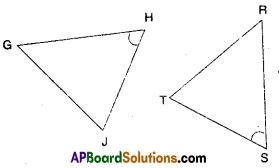Solution:
We need
HG = TS
HJ = TRQuestion 2.
The map given below shows five different villages. Village M lies exactly halfway between the two pairs of villages A and B as well as and P and Q. What is the distance between village A and village P. (Hint: check if ΔPAM ≅ ΔQBM)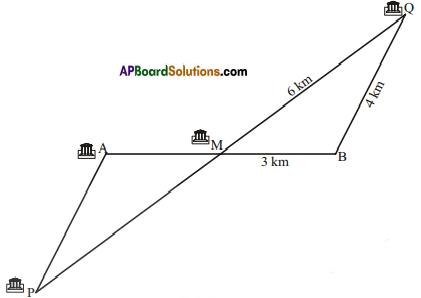Solution:
Given that
AM = MB
PM = MQ
also ∠PMA = ∠QMB
∴ By S.A.S
ΔPMA ≅ ΔQMB
Now PA = BQ
(∵ Corresponding parts of congruent triangles are equal)
∴ PA = 4 km
i.e., Distance between the villages
A and P is 4 km.Question 3.
Look at the pairs of triangles given below. Are they congruent ? If congruent write the corresponding parts.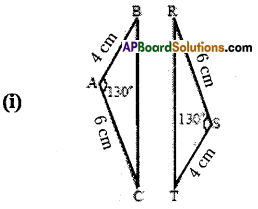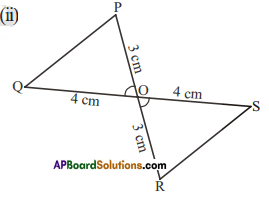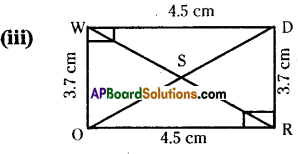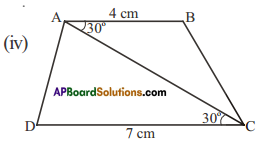Solution:AB=ST
AC = SR (given)
∠A = ∠S
∴ ΔABC ≅ ΔSTR (S.A.S)
Also ∠A = ∠S, ∠B = ∠T, ∠C = ∠RFrom the figure,
FO = RO
OQ = OS
∠POQ = ∠ROS
∴ ΔPOQ ≅ ΔROS (SAS)
Also ∠P = ∠R, ∠Q = ∠S, and PQ = RSFrom the figure, IAPBoardSoIJ
WD = OR
∠W= ∠R
WO = RD
∴ ΔDWO ≅ ΔORD
Also ∠EO = ∠OE; ∠WDO = ∠ROD; ∠WOD = ∠RDOHere the two triangles ΔABC and ΔCDA are not congruent.

Question 4.
Which corresponding sides do we need to know to prove that the triangles are congruent using the SAS criterion?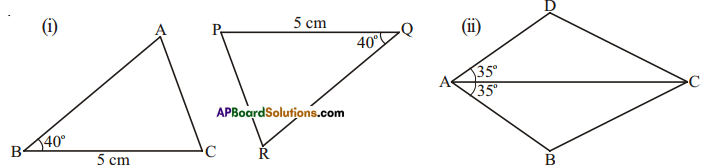Solution:
i) We need to know that AB = QR.
ii) We need to know that AD = AB.